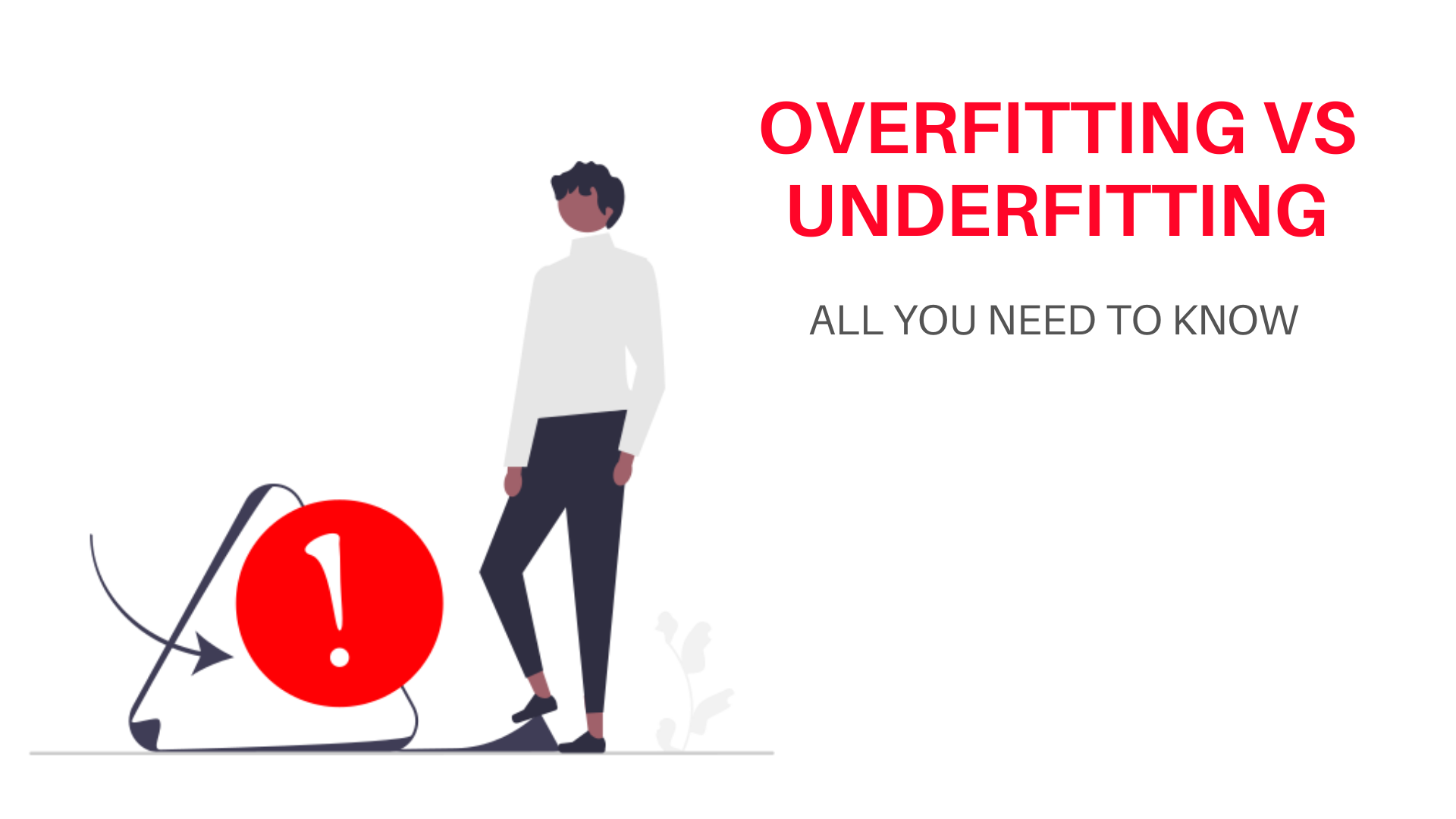# Bias Variance Overfitting Vs Underfitting: All you need to know!Bias Variance Overfitting vs Underfitting is one of the most important metrics you need to understand when you fit your data on any given machine learning model. Understanding it not only helps you prepare a better machine learning model but also can help you improve your model’s performance exponentially by revealing the underlying tendency of the fit of your model.

In this article, we’ll go over understanding each term clearly and interpreting what the bias-variance trade-off really can mean.

## Bias Variance Overfitting vs Underfitting: Understanding each term

### Bias

The difference between the average prediction of our model and the correct value that we are trying to predict is termed, Bias. When a machine learning model boasts of high bias, it always leads to high errors in training and test data.

Some very famous low-bias machine learning algorithms are Decision Trees, k-Nearest Neighbours and Support Vector Machine while high bias machine learning algorithms are Linear Regression, Linear Discriminant Analysis, Logistic Regression.

### Variance

The variability of model prediction for a given value which tells us the spread of our data is called variance. A machine learning model with high variance performs very well on training data but has high error rates on test data.

Some Examples of low variance machine learning algorithms include Linear Regression, Linear Discriminant Analysis, and Logistic Regression. While those high variance machine learning algorithms are Decision Trees, k-Nearest Neighbours, and Support Vector Machines.

One can clearly note that the examples of high bias algorithms are the same as those for low variance and the examples of low bias algorithms are the same as those for a high variance which shows us how one is depending on the other and that it calls for a balance between the two to ensure that the machine learning algorithm neither overfits nor under fits the data.

For more on how bias-variance trade-off works: What is the bias-variance trade-off?

## Overfitting vs Underfitting

In the simplest terms, Bias Variance Overfitting vs Underfitting talks about how one can either consider the noise in a dataset and either be too dependent on the noise to make a very complicated pattern of the dataset that the algorithm is given or to be independent of the noise to make a very simple pattern that does not capture the underlying patterns of the dataset.

Balancing overfitting and underfitting is important to ensure you don’t miss out on the underlying pattern in the data.

In both the cases i.e., overfitting and underfitting, there’s something for you to lose in terms of performance.

### Overfitting

If you are overfitting then your dataset will work tremendously well on the training data but fail miserably on the real-world data or any test data that it hasn’t seen before. In other words, overfitting makes a tailor-made model specifically for the patterns only in the training data and leaves nothing to generalize.

### Underfitting

As far as underfitting is concerned, the model will generalize everything and fail to notice any pattern that is in the data which will make it useless on the training data as well as the test data. The model is just not going to see your data for what it is when you underfit your data.

For more on Overfitting and Underfitting: Overfitting vs Underfitting

## What is the bias variance Overfitting vs Underfitting?

When building machine learning algorithms, data scientists are usually forced to make decisions about the level of bias and variance that can be there in their models. The bias-variance trade-off is well known and explained in basic machine learning courses where the trade-off is very simple: increasing bias decreases the variance, and increasing variance decreases the bias. It is a task for the data scientist to find the correct balance.

In a supervised machine-learning algorithm, the goal is to achieve low bias and variance for getting the most accurate predictions. Data scientists must do this while keeping the underlying possibility of underfitting and overfitting in mind. If a model exhibits small variance and high bias, it will underfit the target variable, while a model with the opposite i.e., high variance and little bias will overfit the target variable.

A model with higher variance may represent the data set accurately but could lead to overfitting to noise or to the otherwise unrepresentative training data. Likewise, a model with high bias may underfit the training data due to a simpler model that overlooks regularities or patterns in the data

## Conclusion

Bias Variance Overfitting vs Underfitting helps in the reduction of the total error of a machine learning model. The Total error of a machine-learning model is the sum of the bias error and variance error making it an essential goal to balance bias and variance, so the model does not underfit or overfit the data.

As the complexity of the model rises or as it continues to increase variance, the bias will decrease. There tends to be a higher level of bias and less variance in a simple machine learning model. To make the models more accurate, a data scientist must find the right balance between bias and variance so that the model minimizes total error and performs without any bias or variance dependence.

Try finding out if your model has more bias or variance and tell us in the comments below what you think about having the right level of bias variance overfitting vs underfitting.

For more such content, check out our website -> Buggy Programmer##### Yash Gupta

An eternal learner, I believe Data is the panacea to the world's problems. I enjoy Data Science and all things related to data. Let's unravel this mystery about what Data Science really is, together. With over 33 certifications, I enjoy writing about Data Science to make it simpler for everyone to understand. Happy reading and do connect with me on my LinkedIn to know more!